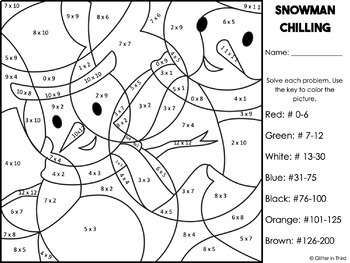Winter Math Color By Number Activities Multiplication FREE3rd - 4th
Subjects
Standards
Resource Type
Formats Included
• PDF
Pages
2 total worksheets

Description

Need a fun and engaging winter math center or your students to review multiplication facts?

These color-by-number worksheets are fun, engaging, and will keep your kiddos focused!

No work needed from you - just print! This is an excellent seasonal math center or morning work.

------------------------------------------------------------------------------------------

Interested in more multiplication resources from Glitter in Third?

Multiples of ten

Multiplication

Multiplication 2-digit by 1-digit

Multiplication fluency

Properties of multiplication

Interactive Notebooks

Multiples of ten

Multiplication fluency

Properties of multiplication

Understanding multiplication

Unknown numbers

Total Pages
2 total worksheets
Included
Teaching Duration
N/A
Report this Resource to TpT
Reported resources will be reviewed by our team. Report this resource to let us know if this resource violates TpT’s content guidelines.

Standards

to see state-specific standards (only available in the US).
Determine the unknown whole number in a multiplication or division equation relating three whole numbers. For example, determine the unknown number that makes the equation true in each of the equations 8 × ? = 48, 5 = __ ÷ 3, 6 × 6 = ?.